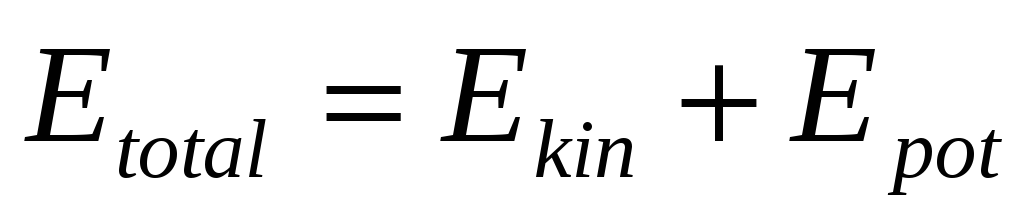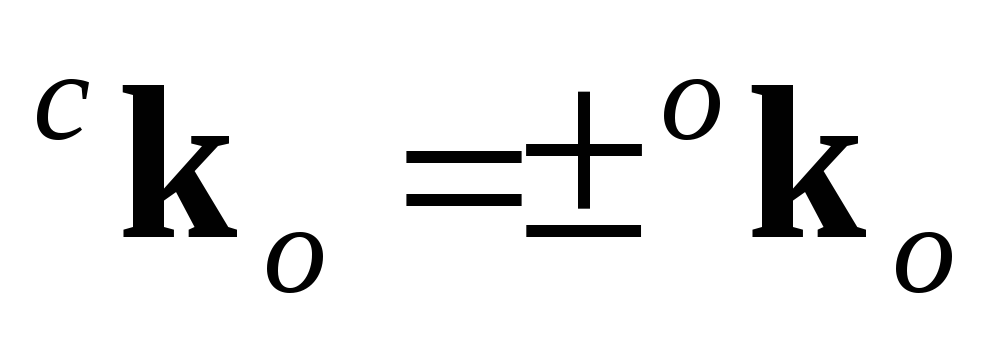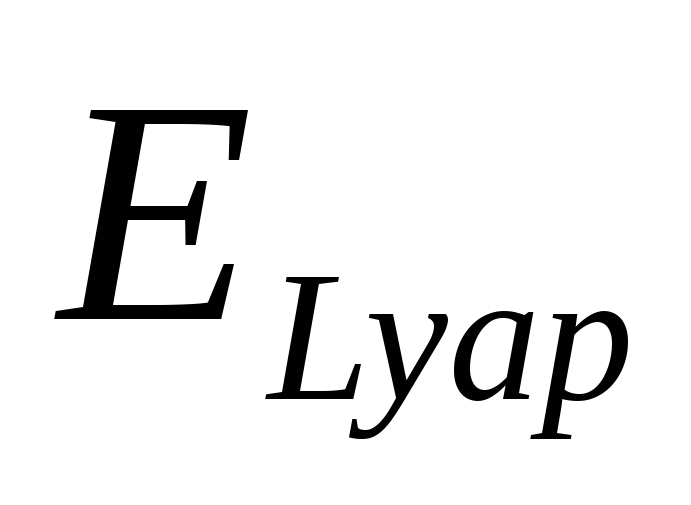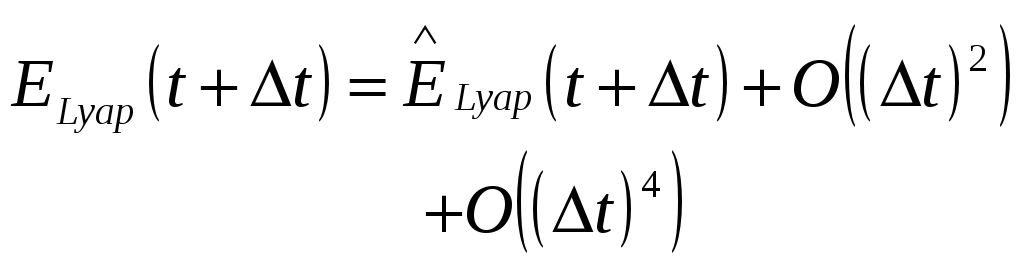# Pedro Tavares, Paulo Tabuada, ‡

 where the ^ stands for prediction. It can be seen from (12) that it is possible to predict the effect that a given control torque will produce on the angular velocity. For this prediction there is only need to know the current angular velocities and attitude, readily available from the attitude determination system. Using the prediction equation (12) and (2) it is possible to choose from the available magnetic moments the one that minimizes the cost function (5), once the geomagnetic field value is available from the magnetometers. Stability study The total energy of satellite is composed of a kinetic term and a potential term,(13) Their sum, the total energy, is constant, since the dissipative forces and torques actuating on a satellite are very weak. By dissipating the kinetic energy, the total energy is also decreased. Since the system is not fully controllable it is not possible to place the satellite in a zero kinetic energy configuration and keep it there because gravity-gradient torques will impose a libration movement converting potencial energy to kinetic energy. All potencial energy is converted to kinetic energy during the libration movement and all kinetic energy is dissipated by the predictive algorithm, therefore the only stable configuration for the satellite is a minimum total energy one (). There is, however, a situation when the predictive algorithm is not capable of dissipating energy, when using on-off actuation and the actuation instants coincide with zero kinetic energy configurations. This situation can be avoided by guaranteeing that the libration movement period, which is a function of the inertia moments and the satellite angular velocity around earth (Chobotov), is different from the actuation period. Having established that a kinetic energy like cost function is enough for stability it is still necessary to show that this minimization method based on a predictive model will work. Consider a Lyapunov candidate functionas defined in (3), the kinetic energy based on (10) may be expressed as:(14) Download 1.52 Mb.Share with your friends: